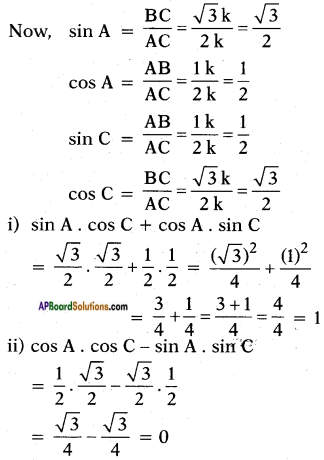# AP SSC 10th Class Maths Solutions Chapter 11 Trigonometry Ex 11.1

AP State Board Syllabus AP SSC 10th Class Maths Textbook Solutions Chapter 11 Trigonometry Ex 11.1 Textbook Questions and Answers.

## AP State Syllabus SSC 10th Class Maths Solutions 11th Lesson Trigonometry Exercise 11.1

### 10th Class Maths 11th Lesson Trigonometry Ex 11.1 Textbook Questions and Answers

Question 1.
In right angle triangle ABC, 8 cm, 15 cm and 17 cm are the lengths of AB, BC and CA respectively. Then, find out sin A, cos A and tan A.
Given that
△ABC is a right angle triangle and Lengths of AB, BC and CA are 8 cm, 15 cm and 17 cm respectively.
Among the given lengths CA is longest.
Hence CA is the hypotenuse in △ABC and its opposite vertex having right angle.
i.e., ∠B = 90°.
With reference to ∠A, we have opposite side = BC = 15 cm
adjacent side = AB = 8 cm
and hypotenuse = AC = 17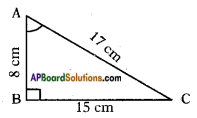sin A = $$\frac{\text { Opposite side of } \angle \mathrm{A}}{\text { Hypotenuse }}$$ = $$\frac{BC}{AC}$$ = $$\frac{15}{17}$$
cos A = $$\frac{\text { Adjacent side of } \angle \mathrm{A}}{\text { Hypotenuse }}$$ = $$\frac{AB}{AC}$$ = $$\frac{8}{17}$$
tan A = $$\frac{\text { Opposite side of } \angle \mathrm{A}}{\text { Adjacent side of } \angle \mathrm{A}}$$ = $$\frac{BC}{AB}$$ = $$\frac{15}{8}$$
∴ sin A = $$\frac{15}{17}$$;
cos A = $$\frac{8}{17}$$
tan A = $$\frac{15}{8}$$Question 2.
The sides of a right angle triangle PQR are PQ = 7 cm, QR = 25 cm and ∠P = 90° respectively. Then find, tan Q – tan R.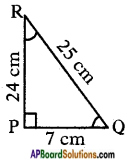Given that △PQR is a right angled triangle and PQ = 7 cm, QR = 25 cm.
By Pythagoras theorem QR2 = PQ2 + PR2
(25)2 = (7)2 + PR2
PR2 = (25)2 – (7)2 = 625 – 49 = 576
PR = √576 = 24 cm
tan Q = $$\frac{PR}{PQ}$$ = $$\frac{24}{7}$$;
tan R = $$\frac{PQ}{PR}$$ = $$\frac{7}{24}$$
∴ tan Q – tan R = $$\frac{24}{7}$$ – $$\frac{7}{24}$$
= $$\frac{(24)^{2}-(7)^{2}}{168}$$
= $$\frac{576-49}{168}$$
= $$\frac{527}{168}$$

Question 3.
In a right angle triangle ABC with right angle at B, in which a = 24 units, b = 25 units and ∠BAC = θ. Then, find cos θ and tan θ.
Given that ABC is a right angle triangle with right angle at B, and BC = a = 24 units, CA = b = 25 units and ∠BAC = θ.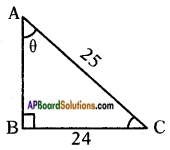By Pythagoras theorem
AC2 = AB2 + BC2
(25)2 = AB2 + (24)2
AB2 = 252 – 242 = 625 – 576
AB2 = 49
AB = √49 = 1
With reference to ∠BAC = θ, we have
Opposite side to θ = BC = 24 units.
Adjacent side to θ = AB = 7 units.
Hypotenuse = AC = 25 units.
Now
cos θ = $$\frac{\text { Adjacent side of } \theta}{\text { Hypotenuse }}$$ = $$\frac{AB}{AC}$$ = $$\frac{7}{25}$$
tan θ = $$\frac{\text { Opposite side of } \theta}{\text { Adjacent side of } \theta}$$ = $$\frac{BC}{AB}$$ = $$\frac{24}{7}$$
Hence cos θ = $$\frac{7}{25}$$ and tan θ = $$\frac{24}{7}$$Question 4.
If cos A = $$\frac{12}{13}$$, then find sin A and tan A.
From the identity
sin2 A + cos2 A = 1
⇒ sin2 A = 1 – cos2 A
= 1 – $$\left(\frac{12}{13}\right)^{2}$$
= 1 – $$\frac{144}{169}$$
= $$\frac{169-144}{169}$$
= $$\frac{25}{169}$$
∴ sin A = $$\sqrt{\frac{25}{169}}$$ = $$\frac{5}{13}$$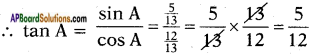∴ sin A = $$\frac{5}{13}$$; tan A = $$\frac{5}{12}$$

Question 5.
If 3 tan A = 4, then find sin A and cos A.
Given 3 tan A = 4
⇒ tan A = $$\frac{4}{3}$$
From the identify sec2 A – tan2 A = 1
⇒ 1 + tan2 A = sec2 A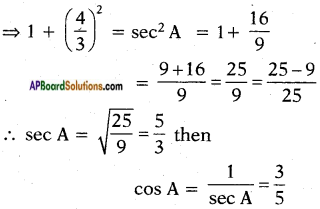If cos A = $$\frac{3}{5}$$ then from
sin2 A + cos2 A = 1
We can write sin2A = 1 – cos2A
= 1 – $$\left(\frac{3}{5}\right)^{2}$$
= 1 – $$\frac{9}{25}$$
⇒ sin2 A = $$\frac{16}{25}$$
⇒ sin A = $$\frac{4}{5}$$
∴ sin A = $$\frac{4}{5}$$; cos A = $$\frac{3}{5}$$Question 6.
In △ABC and △XYZ, if ∠A and ∠X are acute angles such that cos A = cos X then show that ∠A = ∠X.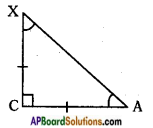In the given triangle, cos A = cos X
⇒ $$\frac{AC}{AX}$$ = $$\frac{XC}{AX}$$
⇒ AC = XC
⇒ ∠A = ∠X (∵ Angles opposite to equal sides are also equal)

Question 7.
Given cot θ = $$\frac{7}{8}$$, then evaluate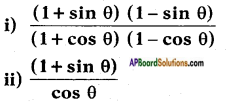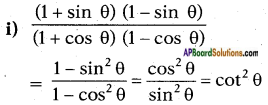cot2 θ = (cot θ)2
= $$\left(\frac{7}{8}\right)^{2}$$ = $$\frac{49}{64}$$ …… (1)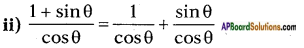= sec θ + tan θ
So cot θ = $$\frac{7}{8}$$
⇒ tan θ = $$\frac{8}{7}$$
⇒ tan2 θ = $$\left(\frac{8}{7}\right)^{2}$$ = $$\frac{64}{49}$$
From sec2 θ – tan2 θ = 1
⇒ 1 + tan2 θ = sec2 θ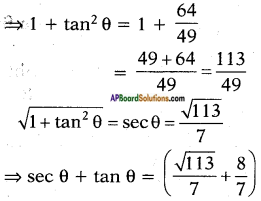Question 8.
In a right angle triangle ABC, right angle is at B, if tan A = √3, then find the value of
i) sin A cos C + cos A sin C
ii) cos A cos C – sin A sin C
Given, tan A = $$\frac{\sqrt{3}}{1}$$
Hence $$\frac{\text { Opposite side }}{\text { Adjacent side }}=\frac{\sqrt{3}}{1}$$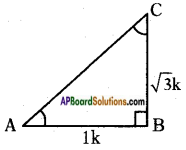∴ AC = $$\sqrt{4 k^{2}}$$ = 2k